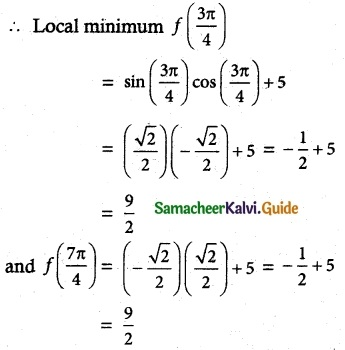Tamilnadu State Board New Syllabus Samacheer Kalvi 12th Maths Guide Pdf Chapter 7 Applications of Differential Calculus Ex 7.6 Textbook Questions and Answers, Notes.

## Tamilnadu Samacheer Kalvi 12th Maths Solutions Chapter 7 Applications of Differential Calculus Ex 7.6

Question 1.
Find the absolute extrema of the following functions on the given closed interval.
(i) f(x) = x² – 12x + 10; [1, 2]
(ii) f(x) = 3x4 – 4x³; [-1, 2].
(iii) f(x)= 6x$$\frac { 4 }{ 3 }$$ – 3x$$\frac { 1 }{ 3 }$$; [-1, 1]
(iv) f(x) = 2 cos x + sin 2x; [0, $$\frac { π }{ 2 }$$ ]
Solution:
(i) f(x) = x² – 12x + 10;
f'(x) = 2x – 12
f'(x) = 0 ⇒ 2x – 12 = 0
x = 6 ∉ (1, 2)
Now, Evaluating f(x) at the end points x = 1, 2
f(1) = 1 – 12 + 10 = -1
f(2) = 4 – 24 + 10 = -10
Absolute maximum f(1) = -1
Absolute minimum f(2) = -10(ii) f(x) = 3x4 – 4x3
f'(x) = 12x3 – 12x2
f'(x) = 0 ⇒ 12x2(x – 1) = 0
⇒ x = 0 or x = 1
[Here x = 0, 1 ∈ [-1, 2]]
Now f (-1) = 4
f(0) = 0
f(1) = -1
f(2) = 16
so absolute maximum = 16 and absolute minimum = -1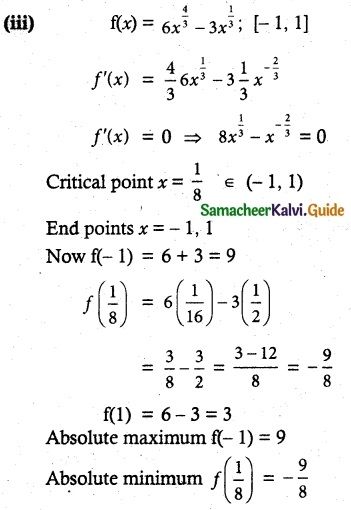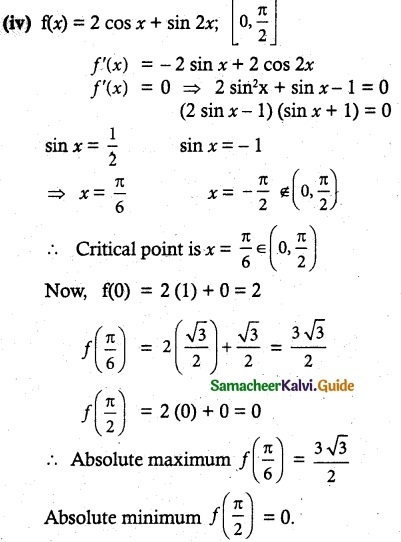Question 2.
Find the intervals of monotonicities and hence find the local extremum for the following functions:
(i) f(x) = 2x³ + 3x² – 12x
(ii) f(x) = $$\frac { x }{ x-5 }$$
(iii) f(x) = $$\frac { e^x }{ 1-e^x }$$
(iv) f(x) = $$\frac { x^3 }{ 3 }$$ – log x
(v) f(x) = sin x cos x+ 5, x ∈ (0, 2π)
Solution:
(i) f(x) = 2x³ + 3x² – 12x
f'(x) = 6x² + 6x – 12
f'(x) = 0 ⇒ 6(x² + x – 2) = 0
(x + 2)(x – 1) = 0
Stationary points x = -2, 1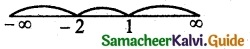Now, the intervals of monotonicity are
(-∞, -2), (-2, 1) and (1, ∞)
In (-∞, -2), f'(x) > 0 ⇒ f(x) is strictly increasing.
In (-2, 1), f'(x) < 0 ⇒ f(x) is strictly decreasing.
In (1, ∞), f'(x) > 0 ⇒ f(x) is strictly increasing.
f(x) attains local maximum as f'(x) changes its sign from positive to negative when passing through x = -2.
Local maximum
f(-2) = 2 (-8) + 3 (4) – 12 (-2)
= -16 + 12 + 24 = 20
f(x) attains local minimum as f'(x) changes its sign from negative to positive when passing through x = 1.
∴ Local minimum f(1) = 2 + 3 – 12 = -7(ii) f(x) = $$\frac { x }{ x-5 }$$
f'(x) = $$\frac { (x-5)(1)-x(1) }{ (x-5)^2 }$$ = –$$\frac { 5 }{ (x-5)^2 }$$
f'(x) = 0, which is absured
But in f(x) = $$\frac { x }{ x-5 }$$
The function is defined only when x < 5 or x > 5
∴ The intervals are (-∞, 5) and (5, ∞)
In the interval (-∞, 5), f'(x) < 0
In the interval (5, ∞), f'(x) < 0
∴ f(x) is strictly decreasing in (-∞, 5) and (5, ∞)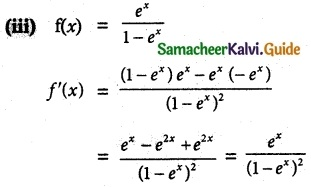When x = 0, f(x) becomes undefined.
∴ x = 0 is an excluded value.
∴ The intervals are (-∞, 0) ∪ (0, ∞) in – (-∞, ∞), f'(x) > 0
∴ f(x) is strictly increasing in (- ∞, ∞) and there is no extremum.

(iv) f(x)= $$\frac { x^3 }{ 3 }$$ – log x
f'(x) = x² – $$\frac { 1 }{ x }$$
f'(x) = 0 ⇒ x³ – 1 = 0 ⇒ x = 1
The intervals are (0, 1) and (1, ∞).
i.e., when x > 0, the function f(x) is defined in the interval (0, 1), f'(x) < 0
∴ f(x) is strictly decreasing in (0, 1) in the interval (1, ∞), f'(x) > 0
∴f(x) is strictly increasing in (1, ∞)
f(x) attains local minimum as f'(x) changes its sign from negative to positive when passing through x = 1
∴ Local minimum
f(1) = $$\frac { 1 }{ 3 }$$ – log 1 = $$\frac { 1 }{ 3 }$$ – 0 = $$\frac { 1 }{ 3 }$$(v) f(x) = sin x cos x + 5, x ∈ (0, 2π)
f'(x) = cos 2x
f'(x) = 0 ⇒ cos 2x = 0
Stationary points
x = $$\frac { π }{ 4 }$$, $$\frac { 3π }{ 4 }$$, $$\frac { 5π }{ 4 }$$, $$\frac { π }{ 4 }$$ ∈x = (0, 2π)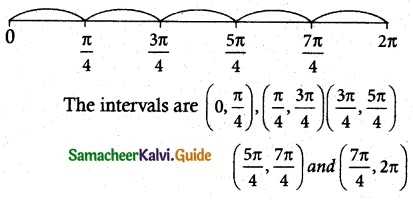In the interval (0, $$\frac { π }{ 4 }$$), f'(x) > 0 ⇒ f(x) is strictly increasing.
In the interval ($$\frac { π }{ 4 }$$, $$\frac { 3π }{ 4 }$$), f'(x) < 0 ⇒ f(x) is strictly decreasing.
In the interval ($$\frac { 3π }{ 4 }$$, $$\frac { 5π }{ 4 }$$), f'(x) > 0 ⇒ f(x) is strictly increasing.
In the interval ($$\frac { 5π }{ 4 }$$, $$\frac { 7π }{ 4 }$$), f'(x) < 0 ⇒ f(x) is strictly decreasing.
In the interval ($$\frac { 7π }{ 4 }$$, 2π), f'(x) > 0 ⇒ f(x) is strictly increasing.
f'(x) changes its sign from positive to negative when passing through x = $$\frac { π }{ 4 }$$ and x = $$\frac { 5π }{ 4 }$$
∴ f(x) attains local maximum at x = $$\frac { π }{ 4 }$$ and $$\frac { 5π }{ 4 }$$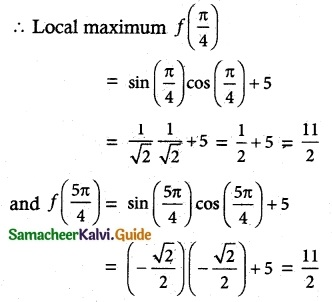f'(x) changes its sign from negative to positive when passing through x = $$\frac { 3π }{ 4 }$$ and x = $$\frac { 7π }{ 4 }$$
∴ f(x) attains local maximum at x = $$\frac { 3π }{ 4 }$$ and x = $$\frac { 5π }{ 4 }$$